### Home > CALC > Chapter 1 > Lesson 1.3.3 > Problem1-129

1-129.
1. Given: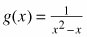Homework Help ✎

1. Find the domain of g(x).

2. Solve for x if g(x) = 0.5.

3. Explain why g(x) does not have an inverse function.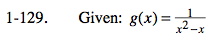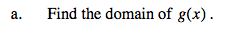Solve for all values for which the denominator is zero. This will make the function undefined. That is, solve x2x = 0.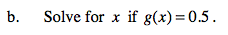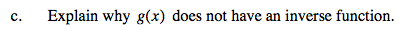Recall that to create the inverse, reflect the original function over the line y = x.